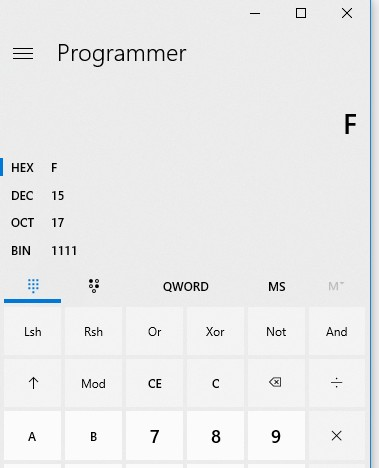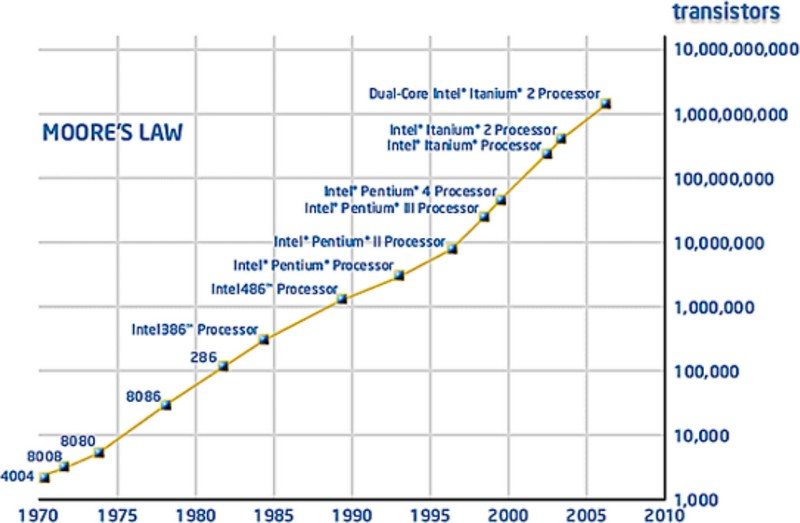# Number - Decimal (base 10) notation

Decimal is a numeral system with a base 10 because it has 10 characters (called digit):

• 0,
• 1,
• 2,
• 3,
• 4,
• 5,
• 6,
• 7,
• 8,
• 9

## Representation

### Whole Number

The usual decimal representation of whole numbers gives every whole number a unique representation as a finite sequence of digits.

### Rational Number

When decimal representation is used for the rational or real numbers, such numbers in general have an infinite number of representations, for example 2.31 can also be written as 2.310, 2.3100000, 2.309999999…, etc., all of which have the same meaning except for some scientific and other contexts where greater precision is implied by a larger number of figures shown.

Discover MoreBit - Binary to Decimal

Binary number to decimal Windows calculator: select the Programmer mode select the BIN format enter the bits copy the DEC value Binary number with Radix to Decimal The binary number...Bit - Numeral system (Base-2) notation - Binary Number

The binary numeral system, or base-2 number system, represents numeric values using two symbols, 0 and 1. Owing to its straightforward implementation in digital electronic circuitry using logic gates,...Computer - Floating-point (Representation and usage)

Computer representations of floating point numbers typically use a form of rounding to significant figures, but with binary numbers. The number of correct significant figures is closely related to the...Number - (Numeral system|System of numeration|Representation of number)

A numeral system (or system of numeration) is a mathematical notation system for expressing numbers using digits (or other symbols). The numeral system gives the context that allows the (digits|symbols)...The radix or base is the number of unique digits, including zero, used to represent numbers in a positional numeral system. For the decimal system the radix is ten, because it uses ten digits: ...Number - Decimal (Hindu–Arabic numeral system)

A decimal number is a number coming from the numeral system base 10. The Hindu–Arabic numeral system, base-10, is the most commonly used system in the world today for most calculations. The prefix...Number - Digit (Numeric symbol)

A digit is a numeric symbol of a numeral system. see The decimal notation (base 10) has 10 digits: 0,1,2,3,4,5,6,7,8,9. When used in combinations, they can represent more number. A whole field...Number - Fixed-point numbers (exact numbers)

A fixed-point number represents a limited-precision rational number that may have a fractional part. These numbers are stored internally in a scaled-integer form, typically in binary but sometimes in...Number - Floating-point (system|notation) - (Float|Double) - Approximate numeric

The term floating point refers to the fact that the number's radix point can float, that is, it can be placed anywhere relative to the significant digits of the number. They are fractional numbers...
Number System - Natural Numbers (cardinal and ordinal numbers) -

A natural number can be used for two purposes: to count (ie describe the size of a set) to order (ie or to describe the position of an element in a sequence). The natural numbers are those used...